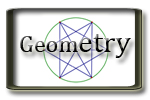G.P. Geometry Unit 1 Basics of Geometry Jump to Unit 1 - 2 - 3 - 4 - 5 - 6 - 7- 8- 9 - 10 - 11 Unit 1 Textbook with Examples and extra problems. Big Ideas Video Tutorials - Lots of video examples

Learning Target

Handouts
Completed Class Notes

Videos and Support Resources

Unit 1 Summary - Good notes to remember
First Day Intro Activity Penny Circles
1.1 Points, Lines, and Planes
 Example 1 Example 2 Example 3 Example 4 Example 5
1.2 Measuring and Constructing Segments
 Example 1 Example 2 Example 3 Example 4
1.3 Using midpoint and distance
 Example 1 Example 2 Example 3 Example 4
1.4 Perimeter and Area in the Coordinate Plane
 Example 1 Example 2 Example 3 Example 4
1.5 Measuring and Constructing
 Example 1 Example 2 Example 3 Example 4 Example 5
1.6 Describing Pairs of Angles
 Example 1 Example 2 Example 3 Example 4 Example 5

Ajacent Angle Video

Ajacent Angles equations

Review

Unit 1 Review

Review Solutions